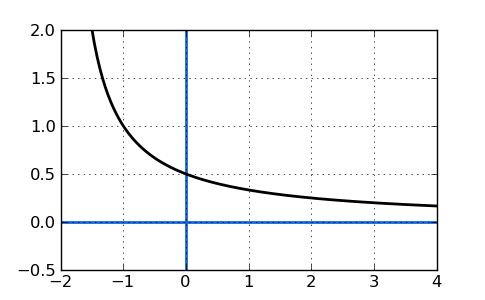# Calculate the limit of an expression

### What is a limit?

A limit is a certain value to which a function approaches. Finding a limit usually means finding what value y is as x approaches a certain number. You would typical phrase it as something like "the limit of a function f(x) is 7 as x approaches infinity. For example, imagine a curve such that as x approaches infinity, that curve comes closer and closer to y=0 while never actually getting there. So, how do we algebraically find that limit? One way to find the limit is by the substitution method.

For example, the limit of the following graph is 0 as x approaches infinity, clearly seen as the graph approaches 0 like so:Now, let's look at a few examples where we can find the limit of real functions:

### Example A

Find the limit of $$f(x) = 4x$$, as x approaches 3.

Steps:

1) Replace x for 3.
2) Simplify.

$$f(x) = 4x$$ becomes $$f(3) = 4(3) = 12$$.

So, the limit of $$f(x) = 4x$$ as x approaches 3 is 12.

In this case the solution was straightforward, because the function not only approaches 12 but goes right through it!

### Example B: Find the limit:

$$\lim_{x\to1} (x^2+5x-3)$$

Follow the same steps as above.

$$x^2+5x-3$$ $$1^2+5*1-3$$ $$=3$$

So, the limit of $$x^2 + 5x - 3$$ as x approaches 1 is 3.

However, the substitution method will not always work. For Example C below, you must factor the numerator first BEFORE applying the substitution method.

### Example C:

$$\lim_{x\to0} \frac{6x^2-7x}{x}$$

If we substitute 0 for x in Example C, we will create division by zero which DOES NOT EXIST or is UNDEFINED. That's is the reason factoring MUST be our first step in this sample. We have to clean it up a bit so there's no division by zero.

Factoring the numerator for x, which is common to both terms, gives us:

$$\frac{6x^2-7x}{x} = \frac{x(6x-7)}{x} = 6x-7$$

We cancelled a factor of x in the numerator and denominator, leaving us with a simple limit:

$$\lim_{x\to0} (6x-7)$$

Now, we can substitute 0 for x to find the limit is -7:

$$\lim_{x\to0} (6x-7) = -7$$

Note: Even though we were able to simplify the function in Sample C by factoring, we can't pretend that it didn't happen. Remember that we were finding the limit as x approached 0, not trying to evaluate the function AT x=0. The function is still undefined at x=0. It does, however, have a limit. Only the simplified version has a solution at x=0. Only after factoring, in some cases, can we then apply substitution to find the limit.

Provided by Mr. Feliz